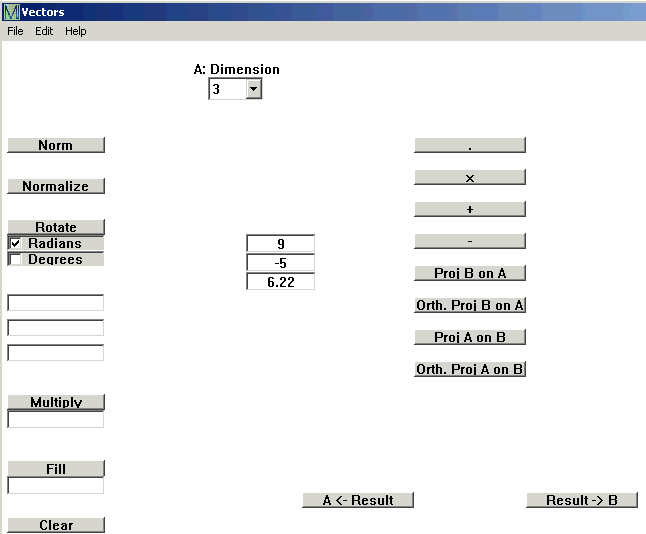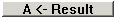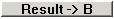# At the top of the window, click Linear Algebra and then Vectors. The Vectors window opens.

Enter the dimension of the first vector under A:Dimensions. This creates boxes in which you type the values of the vector.Here I have entered the vector (9, -5, 6.22).

For a vector with one value repeated often, type the value in the box below fill and click Fill.

If you have a second vector, type B:Dimension. A new column of boxes opens on the right, where you enter the values. Now to calculate

Norm (often called Magnitude or Length or sometimes Absolute Value)

Normalize

Rotate

Multiply (often called Scalar Product)

Dot Product

Cross Product

The result of a calculation appears in the bottom middle of the screen. You can move the result to the left or right side usingorand then do more calculations.